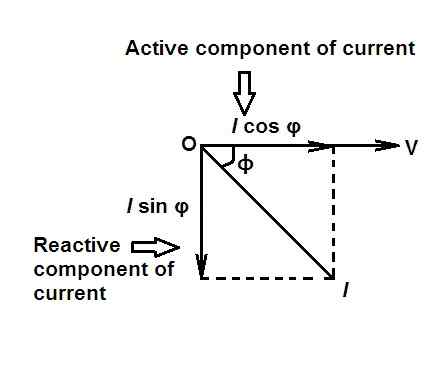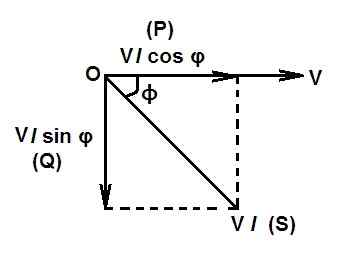# Power Factor in AC Circuit

In DC circuits, the product VI gives the power in watts drawn by the circuit. But in AC circuits, things are different. In AC circuits, the product VI gives the apparent power and not the real power because the voltage and current are not in phase with each other.

# Power Factor in AC Circuit

The power factor in ac circuit may be defined as:

• the cosine of the phase angle between voltage and current i.e. cos φ or
• the ratio of the resistance to impedance cos φ = R/Z
• the ratio of the true to apparent power
i.e. power factor, cos φ = true power/apparent power.

In the case of pure resistive AC circuit, current is in phase with applied voltage i.e.     φ = 0. Therefore, the power factor of the pure resistive circuit (cos φ) is 1.

Whereas in the case of pure capacitive or pure inductive circuit, current is 90o out of phase with circuit voltage i.e. φ = 90o. Therefore, the power factor of the circuit is zero. The power factor of RLC series circuit lies between 0 and 1. The inductive circuits have lagging power factor whereas capacitive circuits have leading power factor.

The power factor can never be greater than unity. Usually the word ‘lagging’ or ‘leading’ is attached with the numerical value of power factor signifying whether the current lags behind or leads the voltage.

## Disadvantages of Low Power Factor in AC Circuit

In AC circuits power factor plays an important role in the power system. Since the power of an AC circuit is given by the relation:

P = V I cos φ
or I = P/ (V cos φ)

It is clear from the above relation, that for a fixed power at a constant voltage, current drawn by the circuit increases with the decrease in power factor. Following are the main disadvantages of low power factor in ac circuit:

• At low power factors conductors have to carry more current for same power, therefore, they require the larger area of cross-section.
• At low power factors, conductors have to carry more current for same power which increases copper losses (I2R) and results in the poor efficiency of the system.
• At low power factors, voltage drop (IR) increases, which results in poor regulation of the system.
• The kVA rating of electrical equipment and machines connected in power system such as transformers, switch gears, alternators etc. will be more at low power factors since it is inversely proportional to power factor (kVA = kW/cos φ.

To improve the power factor (p.f) of an AC circuit a capacitor is connected parallel to the circuit.

## Active and Reactive Component of CurrentThe active component of current is that which is in phase with applied voltage i.e.         I cos φ. It is also known as the watt-full component of current.

Reactive component of current is that which is in quadrature with applied voltage i.e.   I sin φ. It is also known as the watt-less component of current.## Active | Reactive | Apparent Power in AC Circuit

Active Power: The power which is actually consumed in the circuit is called active power or true power. It is denoted by P. The watt-meters indicate the active power of the circuit. The current in phase with the voltage produces true or active power.

Hence, True power, P = voltage x current in phase with voltage

P = VI cos φ

The active power or true power of the circuit is expressed in watts or kilowatts. The true power produces heat in heaters, torque in motors, light in lamps etc. Once this power is used in the circuit, it cannot be recovered.

Reactive Power: The reactive component of current (i.e. I sin φ) when multiplied with circuit voltage results in reactive power. It is denoted by Q.

Hence, Reactive power, Q = VI sin φ

It is expressed in VAR (reactive volt amperes) or KVAR (kilovolt-ampere reactive). The reactive power does not do any useful work in the circuit. It is the power which is supplied by the source during the first quarter cycle and returns back to the source during the next quarter cycle. However, it determines the power factor of the circuit.

Apparent Power (S): The product of RMS values of current and voltage is called the apparent power and is measured in VA (volt-amperes) or KVA (kilo-volt ampere).

Hence, Apparent power, S = VI

It is so called because it appears that product of current and voltage is power. But in AC circuits, except pure resistive circuits, there is usually a phase difference between current and voltage so that VI does not give true power or active power.

# Why are electrical Transformers and Alternators rated in KVA ?

It is so because the maximum apparent power to be carried out by these electrical machines has a direct influence on its physical size.

• As the operating current of an electrical machine increases, we have to employ thicker conductor and size of a machine increases.
• As the line voltage becomes higher, we have to provide greater insulation which results in increase in size of the machine.

Thus the size of an electrical equipment depends upon the product of VI. That is why these electrical machines are rated in VA or KVA.

Also, the kVA is fixed whereas KW is variable in the case of AC machines. This factor also favors the rating of AC machines in kVA very much.

AC Fundamenatls I All Posts

© http://www.yourelectricalguide.com/ power factor in ac circuit.